# Charge Conservation

The fundamental idea of charge conservation is contained in Maxwell's Equations. If we take the divergence of the differential form of Ampere's law: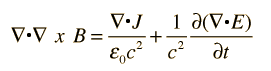The first term above is zero by identity, and using Gauss' law:the result is: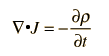The implication here is that the current through any enclosed surface is equal to the time rate of charge within the surface. This is an important test of Maxwell's equations since all experimental evidence points to charge conservation.

 Maxwell's Equations
Index

Maxwell's equations concepts

 HyperPhysics***** Electricity and Magnetism R Nave
Go Back

# The Wave Equation

Maxwell's Equations contain the wave equation for electromagnetic waves. One approach to obtaining the wave equation:

1. Take the curl of Faraday's law: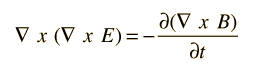2. Substitute Ampere's law for a charge and current-free region: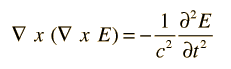This is the three-dimensional wave equation in vector form. It is hard to visualize in this form. It looks more familiar when reduced a plane wave with field in the x-direction only: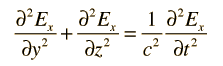Since the electric field is in the x-direction only, the propagation is perpendicular to the x-axis and can be in any direction in the yz plane, depending upon the values of the derivatives. This equation is in the general form of the two-dimensional wave equation.

 Discussion of wave equation Maxwell's Equations
Index

Maxwell's equations concepts

 HyperPhysics***** Electricity and Magnetism R Nave
Go Back